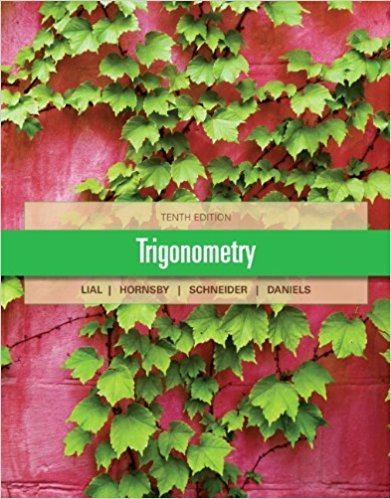×
×

# Answer: Use the appropriate reciprocal identity to findISBN: 9780321671776 231

## Solution for problem 10 Chapter 1.4

Trigonometry | 10th Edition

• Textbook Solutions
• 2901 Step-by-step solutions solved by professors and subject experts
• Get 24/7 help from StudySoup virtual teaching assistantsTrigonometry | 10th Edition

4 5 1 293 Reviews
22
3
Problem 10

Use the appropriate reciprocal identity to find each function value. Rationalize denominators when applicable. See Example 1. sin u, given that csc u = 224 3

Step-by-Step Solution:
Step 1 of 3

Chapter 1: Sections 1-6 1.1 What is a Function A function is a rule that takes certain numbers as inputs and assigns to each a definite output number. Domain: the set of all input numbers Range: the set of all output numbers Independent Variable: input Dependent Variable: output Interval Notation: Closed Intervals: a ⦤ t ⦤b and is written [a,b] Open Intervals: a <...

Step 2 of 3

Step 3 of 3

##### ISBN: 9780321671776

This full solution covers the following key subjects: . This expansive textbook survival guide covers 59 chapters, and 3747 solutions. This textbook survival guide was created for the textbook: Trigonometry, edition: 10. Trigonometry was written by and is associated to the ISBN: 9780321671776. Since the solution to 10 from 1.4 chapter was answered, more than 232 students have viewed the full step-by-step answer. The full step-by-step solution to problem: 10 from chapter: 1.4 was answered by , our top Math solution expert on 01/11/18, 01:35PM. The answer to “Use the appropriate reciprocal identity to find each function value. Rationalize denominators when applicable. See Example 1. sin u, given that csc u = 224 3” is broken down into a number of easy to follow steps, and 26 words.

Unlock Textbook Solution